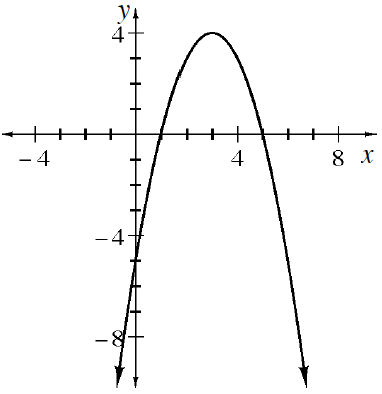### Home > APCALC > Chapter 1 > Lesson 1.4.2 > Problem1-161

1-161.

The parabola $y = -(x - 3)^2 + 4$ is graphed below. Use four trapezoids of equal width to approximate the area under the parabola for $1 ≤ x ≤ 5$. Is this area an overestimate or an underestimate of the true area under the parabola? Explore this using the Estimating Area Under a Curve eTool (Desmos). Homework Help ✎Use the eTool below to visualize the flag.
Click the link at right for the full version of the eTool: Estimating Area Under a Curve eTool